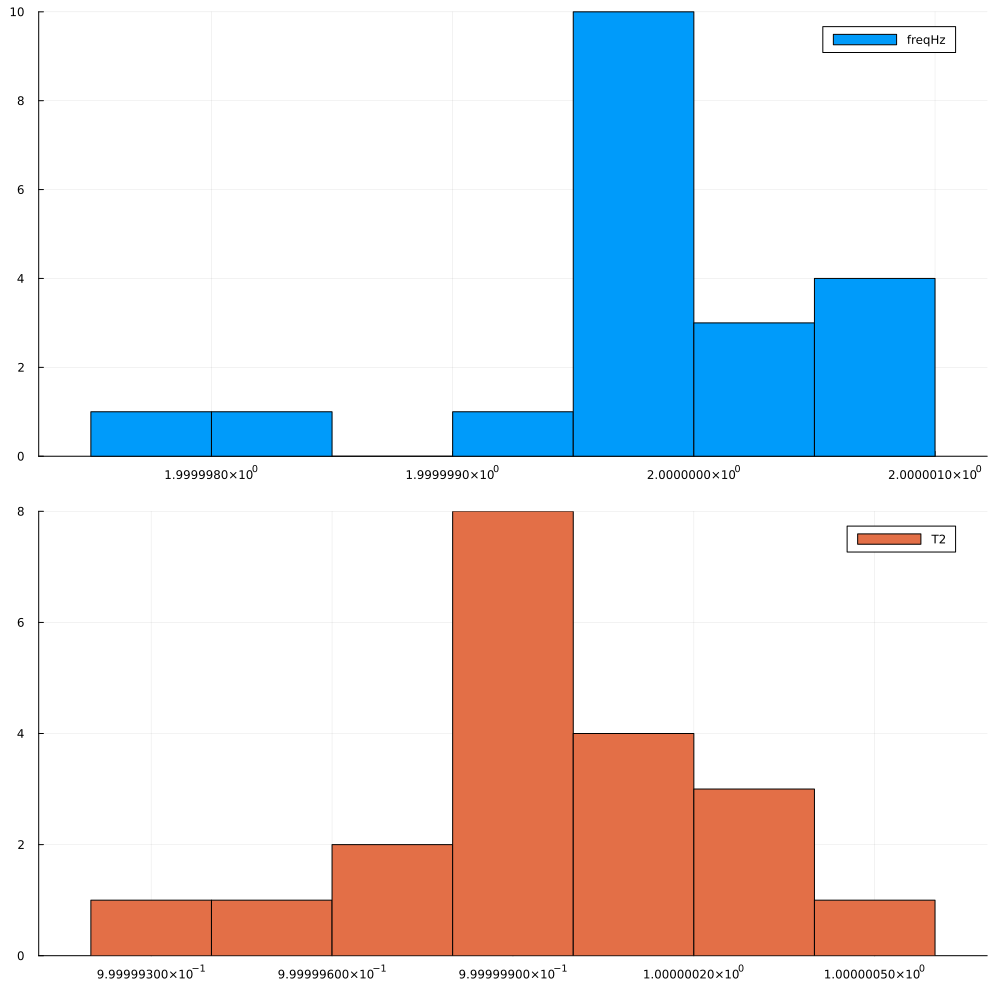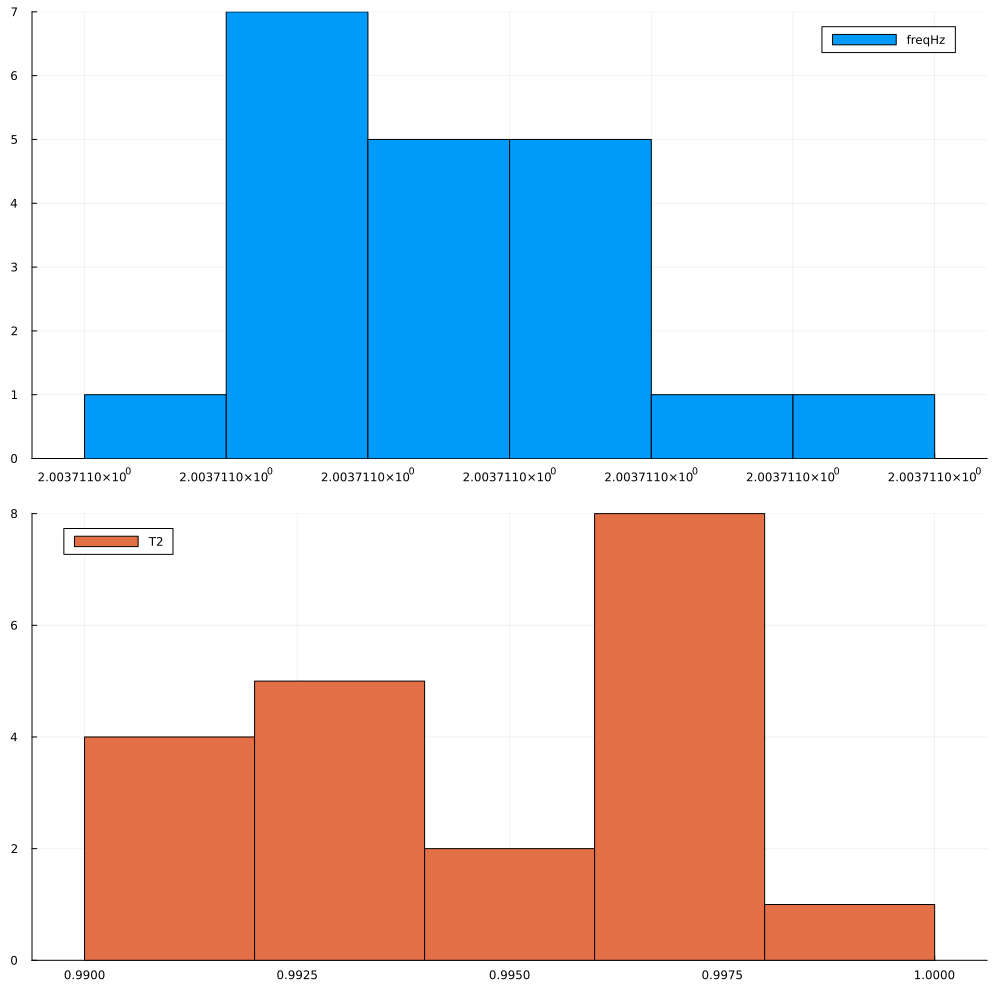# Parametric Uncertainty Quantification with Functional Mockup Units (FMUs)

This example demonstrates how use Functional Mockup Units (FMUs), following Functional Mockup Interface v2.0, to perform parametric uncertainty quantification with JuliaSimModelOptimizer.

The example is based on a Coupled-Clutches model that is available as an FMU. This Coupled-Clutches FMU is a model of a clutch system with three clutches.

## Julia Environment

Let us load the required packages.

using FMI
using DataFrames, CSV
using JuliaSimModelOptimizer
using Plots

## Model Exchange Mode

Let us first demonstrate the use of the Coupled-Clutches FMU in Model Exchange mode.

We start of by loading the FMU using FMI.jl - an open-source julia package which enables working with FMUs. We specify the type of the FMU as FMI.fmi2TypeModelExchange since we intend to simualate the FMU in Model Exchange mode.

fmu = fmiLoad(joinpath(@__DIR__, "../assets/CoupledClutches_ME.fmu"); type = FMI.fmi2TypeModelExchange)
Model name:        CoupledClutches
Type:              0

We load the data from a CSV file using the DataFrames.jl package. The data is a time series of the states of the system, such as the angular velocity and acceleration of the inertias.

data = CSV.read(joinpath(@__DIR__, "../assets/CoupledClutches_data.csv"), DataFrame)
5×9 DataFrame
RowtimestampJ1.phiJ1.phi.derJ2.phiJ2.phi.derJ3.phiJ3.phi.derJ4.phiJ4.phi.der
Float64Float64Float64Float64Float64Float64Float64Float64Float64
10.00.010.00.00.00.00.00.00.0
20.010.09955279.915840.0004993590.0997420.00.00.00.0
30.020.1984219.862870.001989630.1979210.00.00.00.0
40.030.2969059.838220.00444740.2929890.00.00.00.0
50.040.3952629.83650.007834020.3834460.00.00.00.0

### Experiment Setup

We next use the data to setup an experiment. We specify the time span of the Experiment and the relative tolerance of the solver. We also specify the InverseProblem of interest to us where we specify the parameters we want to recover and their bounds.

ex = Experiment(data, fmu; tspan = (0.0, 1.5), reltol = 1e-5)
invprob = InverseProblem(ex, fmu, ["freqHz" => (0.05, 3), "T2" => (0.2, 1.5)])
InverseProblem for Functional Mock-up Unit 2.0 with one experiment optimizing 2 parameters.


### Run Parametric Uncertainty Quantification

We now run the parameteric uncertainty quantification using the parametric_uq function. We specify the InverseProblem and the StochGlobalOpt algorithm. We also specify the sample size for the analysis.

results = parametric_uq(invprob, StochGlobalOpt(; maxiters = 3000), sample_size = 20)
Parametric uncertainty ensemble of length 20 computed in 7 minutes and 55 seconds.
┌────────┬──────────┐
│ freqHz │       T2 │
├────────┼──────────┤
│    2.0 │      1.0 │
│    2.0 │      1.0 │
│    2.0 │      1.0 │
│    2.0 │      1.0 │
│    2.0 │ 0.999999 │
│    2.0 │      1.0 │
│    2.0 │      1.0 │
│    2.0 │      1.0 │
│    2.0 │      1.0 │
│    2.0 │      1.0 │
│    2.0 │      1.0 │
│    2.0 │      1.0 │
│    2.0 │      1.0 │
│    2.0 │      1.0 │
│    2.0 │      1.0 │
│    2.0 │      1.0 │
│   ⋮    │    ⋮     │
└────────┴──────────┘
4 rows omitted

Note

Note that we need a sample size of at least 5000 to get a good result and this low sample size is just for demonstration. Same with the number of maxiters parameter of StochGlobalOpt.

### Visualize the results

We can see the results of the analysis by plotting its histogram.

plot(results, layout=(2,1), bins = 8, show_comparision_value = false, legend = :best, size = (1000,1000))We can see the distribution for both the parameters is concentrated near their true values.

## Co-Simulation Mode

The steps for using FMUs in Co-Simulation mode are similar to those for Model Exchange mode. The only difference is that we specify the type of the FMU as FMI.fmi2TypeCoSimulation and we use the DiscreteExperiment instead of the Experiment to setup the experiment as Co-Simulation FMUs are discrete time models and specify the step size (here 1e-2) compulsorily.

fmu = fmiLoad(joinpath(@__DIR__, "../assets/CoupledClutches_CS.fmu"); type = FMI.fmi2TypeCoSimulation)
de = DiscreteExperiment(data, fmu, 1e-2; tspan = (0.0, 1.5))
invprob = InverseProblem(de, fmu, ["freqHz" => (0.05, 3), "T2" => (0.2, 1.5)])
InverseProblem for Functional Mock-up Unit 2.0 with one experiment optimizing 2 parameters.


### Run Parametric Uncertainty Quantification

The rest of the code remains the same as for the Model Exchange FMU.

results = parametric_uq(invprob, StochGlobalOpt(; maxiters = 3000), sample_size = 20)
Parametric uncertainty ensemble of length 20 computed in 2 minutes and 24 seconds.
┌─────────┬──────────┐
│  freqHz │       T2 │
├─────────┼──────────┤
│ 2.00371 │ 0.997265 │
│ 2.00371 │ 0.990419 │
│ 2.00371 │ 0.992624 │
│ 2.00371 │ 0.996264 │
│ 2.00371 │ 0.996497 │
│ 2.00371 │ 0.997826 │
│ 2.00371 │ 0.994068 │
│ 2.00371 │ 0.997419 │
│ 2.00371 │  0.99467 │
│ 2.00371 │ 0.992008 │
│ 2.00371 │ 0.996819 │
│ 2.00371 │ 0.992827 │
│ 2.00371 │ 0.991196 │
│ 2.00371 │  0.99167 │
│ 2.00371 │ 0.992533 │
│ 2.00371 │  0.99772 │
│    ⋮    │    ⋮     │
└─────────┴──────────┘
4 rows omitted


### Visualize the results

We can see the results of the analysis by plotting its histogram.

plot(results, layout=(2,1), bins = 8, show_comparision_value = false, legend = :best, size = (1000, 1000))Again, we can see the distribution for both the parameters is concentrated near their true values.